# ARIMA Time Series Forecasting Model with Python

We’ll build an ARIMA Model using Python to predict house sale price. Here is the source code and the dataset.

And now let’s get started

# The Data

Our data comes from website Kaggle.com and it contains the general information such as “area”, “no_of_crimes” and so on. We need the average price of selling the houses from area. We will visualize the data to see what we are working with.

# The Code

Here are the main modules for data visualization and math manipulation. For forecasting model we use statsmodels.

Let’s load the dataset into a Pandas data frame and prepare it for work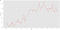Date || Average Price

Its really important to see if time series is stationary or non-stationary. If time series is stationary and it have a special behavior at the given time, then it can have the same behavior at some later point in time. Most statistical modelling methods require the time series to be stationary.

There are two ways to see if the given data is stationary or not: Rolling statistic and Augmented Dickey-Fuller Test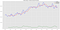Plotted mean against STD

The rolling mean and rolling standard deviation increase over time. This is the point where we can understand that our time series is non-stationary.

From the ADF Statistic we can conclude that the time series is non-stationary. How? Because the ADF Statistic is far from the critical values and the P-Value is greater than the threshold. The simplest way to lower the rate is to take the log of the dependent variable.

As we can see the ADF Statistic is far from the critical value. In conclusion, the time series is non-stationary.

Taking the log of the dependent variable is a simple way to lower the rate at which rolling mean increases.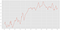logarithm graph

In that case, we’ll create a function to run 2 different tests to determine when a given time series is stationary

Now let’s use our function and render our time series to stationary. In this case we’ll subtract the rolling mean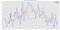Plotted mean against STD

Now the rolling mean and standard deviation are almost horizontal. Which means that ADF Statistic is close enough to the demanding values. And now the time series is stationary.

There is another method, which is exponential decay, that will help us to transform the time series: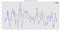Plotted mean against STD

As we can see the exponential decay achieves lower accuracy than subtracting the rolling mean. Well, it’s still better than the original. Anyway this isn’t essential but still has it’s usage.

And now let’s try the last method to see whether it has the better solution. The time shifting replace every point by one that anticipate it.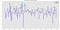Plotted mean against STD

As we can see the time shifting performance is worse than subtracting the rolling mean, but it’s more stationary.

# ARIMA

Auto-regressive integrated moving average (ARIMA) model is a generalization of an auto-regressive moving average (ARMA) model. Both of these models are fitted to time series data either to better understand the data or to predict future points in the series (forecasting). ARIMA models are applied in some cases where data show evidence of non-stationarity, where an initial differencing step (corresponding to the “integrated” part of the model) can be applied one or more times to eliminate the non-stationarity.

The AR part of ARIMA indicates that the evolving variable of interest is regressed on its own lagged (i.e., prior) values.

The MA part indicates that the regression error is actually a linear combination of error terms whose values occurred contemporaneously and at various times in the past.

The I (for “integrated”) indicates that the data values have been replaced with the difference between their values and the previous values (and this differencing process may have been performed more than once). The purpose of each of these features is to make the model fit the data as well as possible.

Non-seasonal ARIMA models are generally denoted ARIMA(p,d,q) where parameters p, d, and q are non-negative integers, p is the order (number of time lags) of the auto-regressive model, d is the degree of differencing (the number of times the data have had past values subtracted), and q is the order of the moving-average model. Seasonal ARIMA models are usually denoted ARIMA(p,d,q)(P,D,Q)m, where m refers to the number of periods in each season, and the uppercase P,D,Q refer to the auto-regressive, differencing, and moving average terms for the seasonal part of the ARIMA model.

When two out of the three terms are zeros, the model may be referred to based on the non-zero parameter, dropping “AR”, “I” or “MA” from the acronym describing the model. For example, ARIMA(1,0,0) is AR(1), ARIMA(0,1,0) is I(1), and ARIMA (0,0,1) is MA(1).

Now we’ll create and fit an ARIMA model with AR = 2, Difference = 1, and MA = 2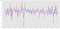decomposition model

Now let’s see how the model fits the time series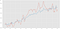predictions ARIMA

Mentioning that our data is going every month for 10 years and want to forecast the house price for the next 5 years, we’ll use the next calculus:

(10 x 10) + (10 x 5) = 150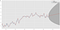forecasting plot

There is a collection of techniques for manipulating the variables that depend on time. ARIMA is the algorithm that can help us to improve to accurately predict the future values.